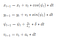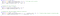# Self-Driving Car Nanodegree Program Term 2 Project MPC Controller

In this project I have implemented a Model Predictive Control (MPC) algorithm to drive the car around the track in C++. The algorithm uses a Kinematic model to predict the car future trajectory and optimizes the actuation inputs to minimize a cost function. The algorithm also handles a 100 millisecond latency between actuation commands.

# Kinematic Models

Kinematic models are simplifications of dynamic vehicle motion models that ignore tire forces, gravity, and mass. At low and moderate speeds kinematic models often approximate actual vehicle dynamics and hence, can be used to test algorithms for self driving cars.Basic Equations of the Kinematic model

# MPC Controller

The implemented MPC model drives the car around the track in the simulator. The model takes as input the state of the vehicle <x,y,v> and the errors <cet,epsi>. The controller controls the steering angle and throttle of the vehicle. The algorithm constantly re-evaluates given the input state to find the optimal actuations and minimize a cost function. It predicts the next points and tries to find the optimal actuations using the above kinematic model. The solver also takes constraints as well as the cost function and finds the optimal actuation.

# Timestep Length and Elapsed Duration

The parameters N and dt describe the perdition horizon for which the model looks ahead and predicts the impact of its actuation in the future. These parameters impact the computational complexity of the model and how it will respond to changes. Setting a very large N results in more computations and a slower model. The chosen values where 10 for N and 0.1 for dt. These values performed reasonably well for the given problem. In addition, when not considering the latency different values can also work well. Some of the values that I tried were N=25,dt=0.2 and N=10,dt=0.01; the latter values do not allow the vehicle to predict too far into the future which in non-ideal cases is problematic. For example, in sharp turns in the simulation the car experienced a zig-zag effect.

# Polynomial Fitting and MPC Preprocessing

The overall process of fitting a polynomial to the track waypoints involved mapping between global and vehicle coordinates and vice versa. The waypoints are given in a global coordinate system. A polynomial can be fitted on those points, however, this introduces complexities in finding the correspondence with vehicle coordinates. A simpler solution is to map the global waypoint coordinates to vehicle coordinates, thus effectively turning the vehicle to the origin of reference. In doing so the position of the vehicle effectively becomes (0,0) and its orientation becomes 0. The coefficients of the fitted polynomial are found and the vehicle state is passed to the MPC. The MPC finds actuator values based on the following cost function with weighted objectives:Implementation of the Cost function in C++ using the Ipopt library

The actuators found by the MPC are given back to the simulator. A final pre-processing step involved normalizing the steering angle by dividing with rad(25) to shift the values between [-1 1].

# Model Predictive Control with Latency

The way that I solved the latency problem was to set the dt to be equivalent to the latency time. In doing so the model was able to predict the next state in the time needed for the actuations to be applied. N was also kept reasonably low for computation efficiency. In the end, N was set to 10 and dt to 0.1. Interestingly, the car was also able to drive the tack with parameters N=5 and dt=0.05, However, it was reckless and in some cases wondered outside the track before going back in again.

# Video Demonstration

PhD in Computer Engineering, Self-Driving Car Engineering Nanodegree, Computer Vision, Visual Perception and Computing

## More from Christos Kyrkou

PhD in Computer Engineering, Self-Driving Car Engineering Nanodegree, Computer Vision, Visual Perception and Computing